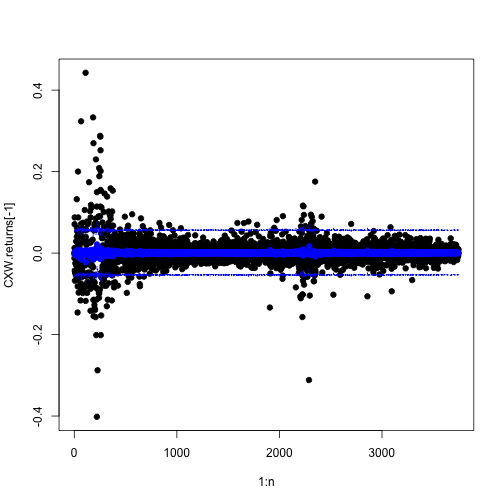# Simulations Encore: More Uses for Simulations

36-350
24 November 2014

### Agenda

• Simulations instead of probability calculations
• Simulations as models
• Building simulations
• Live coding of a simulation model under the direction of the class

### Previously

• Drawing independent random variables
• Making random variables dependent on each other
• Using Monte Carlo to short-cut integration

### vs. Probability Calculations

• Monte Carlo: $\int{g(x) p(x) dx} \approx \frac{1}{n}\sum_{i=1}^{n}{g(X_i)} ~ \mathrm{when} ~ X_i \sim p$
• Not just integrals: | Probability of anything $$\approx$$ how often does it happen in the simulation

### Example: Prediction Intervals

Stock returns of the Corrections Corp. of America:

require(pdfetch)
# Corrections Corp. of America
from=as.Date("2000-01-01",
to=as.Date("2014-09-30")))
CXW.returns <- na.omit(as.vector(diff(log(CXW\$CXW))))


### Example: Prediction Intervals

Regress tomorrow's returns on today's:

CXW.ar <- lm(CXW.returns[-1] ~ CXW.returns[-length(CXW.returns)])
coefficients(CXW.ar)

                      (Intercept) CXW.returns[-length(CXW.returns)]
0.0005609                        -0.0515824


### Example: Prediction Intervals

n <- length(CXW.returns)-1
plot(1:n, CXW.returns[-1],pch=19)
points(1:n, fitted(CXW.ar), col="blue",pch=19)### Example: Prediction Intervals

Could work out prediction intervals from theory, if they were Gaussian … or just simulate

prediction.interval <- function(model, confidence=0.95, n=1e3) {
residuals <- residuals(model)
sim <- sample(residuals, size=n, replace=TRUE)
lower.limit <- (1-confidence)/2
upper.limit <- 1-lower.limit
return(quantile(sim, c(lower.limit, upper.limit)))
}
(CXW.interval95 <- prediction.interval(CXW.ar))

    2.5%    97.5%
-0.05413  0.05586


### Example: Prediction Intervals

plot(1:n, CXW.returns[-1],pch=19)
points(1:n, fitted(CXW.ar), col="blue",pch=19)
lines(1:n, fitted(CXW.ar)+CXW.interval95, lty="dashed", col="blue")
lines(1:n, fitted(CXW.ar)+CXW.interval95, lty="dashed", col="blue")### Example: Prediction Intervals

(This example is slightly defective since it doesn't include parameter uncertainty; we'll cover that in 402 when we do bootstrapping)

### Simulations as Models

• Sometimes the only convenient way to specify the statistical model is as a simulation
• Lots of details, no simplifying math
• Running the simulation is then how we see what the model does at given parameters
• Fit by matching simulation output to data

### Example: Antibiotics Again

• Doctors have either adopted or they haven't
• Every day, two random doctors meet
• If one has adopted but the other hasn't, the hold-out adopts with probability $$p$$
• Look at number of adoptions over time

This was originally a model of disease spread, but now the “disease” is adopting a new drug

[Break for live coding under the direction of the class]

### Example: Antibiotics Again

Code written by section 1:

sim_doctors_1 <- function(num.doctors, num.days, initial_doctors, p) {
# Remember to set up all_doctors
all_doctors <- 1:num.doctors
# Remember to set up has_adopted as binary vector
# Set some doctors to have initially adopted
# initial_doctors are indices of doctors who are using as of day 1
for (today in 1:num.days) {
# pull two random doctors
todays_doctors <- sample(all_doctors,size=2,replace=FALSE)
# check that one has adopted and the other hasn't
}
}
}


### Example: Antibiotics Again

Code written by section 2:

sim_doctors_2 <- function(num.doctors,num.meetings,starting_adopters, prob) {
# vector to keep track of which doctors have adopted
# Set some to have adopted initially
# matrix to keep track of adoptions over time
for (meeting in 1:num.meetings) {
# select 2 doctors at random
meeting_pair <- sample(1:num.doctors,size=2)
# check whether exactly one selected doctor has adopted
# With probability prob., update the vector of adopters
if(rbinom(n=1,size=1,prob=prob)) { has_adopted[meeting_pair] <- TRUE }
}
}
}


### Example: Antibiotics Again

sim2 <- sim_doctors_2(num.doctors=10,num.meetings=10,starting_adopters=0.1,prob=1.0)
dim(sim2)

 10 10

• Start small for debugging
• Making transmission always successful lets us see about updating

### Example: Antibiotics Again

plot(colSums(sim2),xlab="Meeting",ylab="Number of adoptees")### Example: Antibiotics Again

Maybe not that small? With only 10 time-steps it's pretty likely we never pick the one initial adoptee

sim2.1 <- sim_doctors_2(num.doctors=10,num.meetings=100,starting_adopters=0.1,prob=1.0)### Example: Antibiotics Again

sim.big <- sim_doctors_2(num.doctors=1000,num.meetings=10000,starting_adopters=0.01,prob=0.5)Elapsed time from starting to code to final figure: 20 minutes

### Example: Antibiotics Again

• These logistic or sigmoid curves are very characteristic of actual product-adoption curves, and of a lot of epidemiology
• This is the “susceptible-infectious” (SI) model Lots of variants
• susceptible-infectious-susceptible (SIS), susceptible-infectious-recovered (SIR)
• multiple stages of infectiousness
• competing infections
• can only transmit to network neighbors, not random pairing
• etc., etc.

### Summary

• When we don't have exact probability formulas or they don't apply, we can simulate to get arbitrarily-good approximations
• If we can describe the process of our model, we can set up a simulation of it